# Electrical Circuit Components resistors electrical resistance Resistance opposes

• Slides: 13Electrical Circuit Components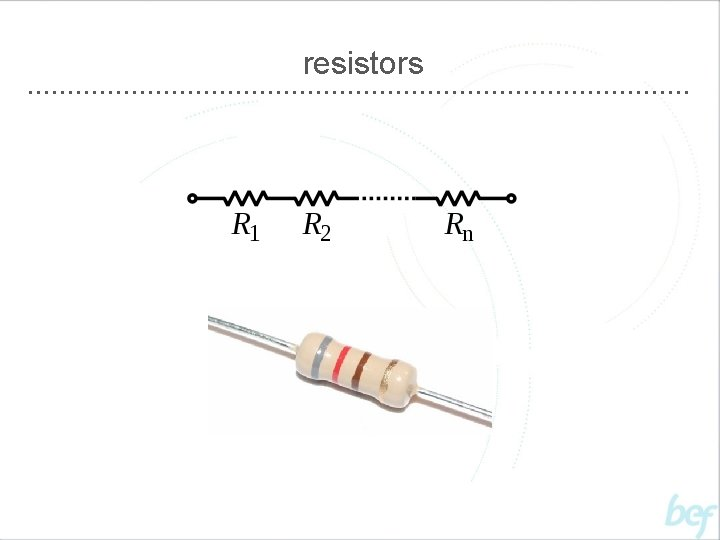resistorselectrical resistance • Resistance opposes the flow of current through a material • It is a property of a material and can be described as that material’s “resistivity” • Resistance = Voltage / Current [R=V/I] • Resistance is measured in Ohms • Conductance (or conductivity) is the opposite of resistance (or resistivity)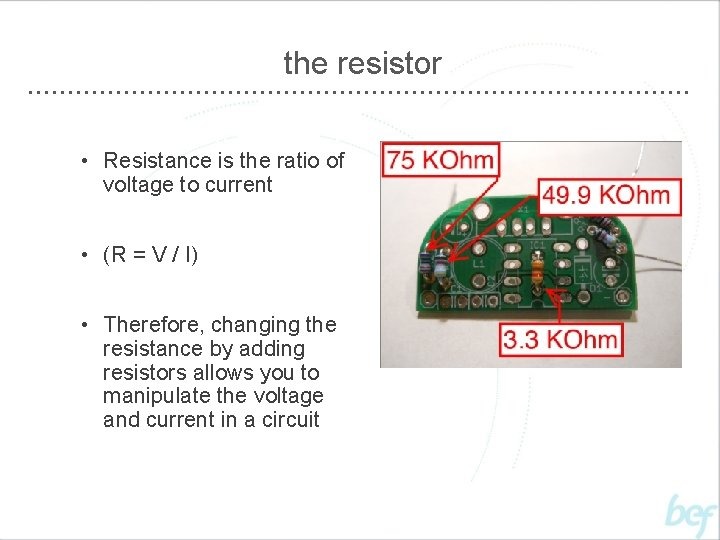the resistor • Resistance is the ratio of voltage to current • (R = V / I) • Therefore, changing the resistance by adding resistors allows you to manipulate the voltage and current in a circuitcapacitors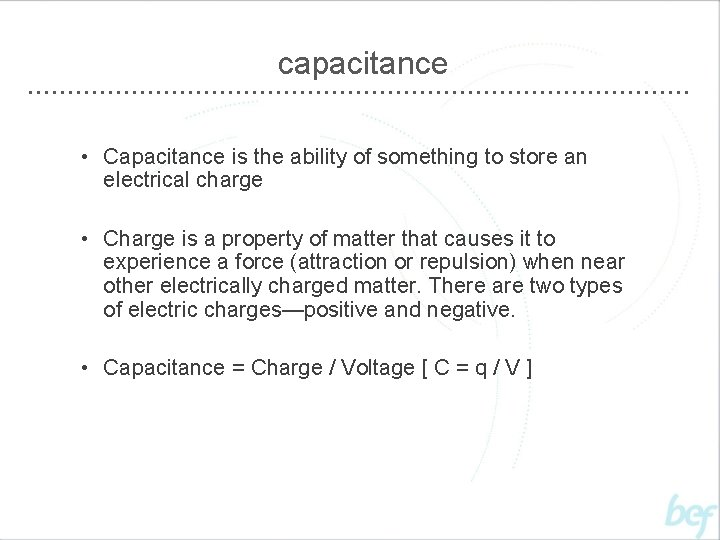capacitance • Capacitance is the ability of something to store an electrical charge • Charge is a property of matter that causes it to experience a force (attraction or repulsion) when near other electrically charged matter. There are two types of electric charges—positive and negative. • Capacitance = Charge / Voltage [ C = q / V ]the capacitor • Capacitors are used in electrical circuits to store energy for later releaseinductorsinductance • Inductance is the process by which a change in current creates voltage • It is a property of a conductor whereby a voltage is “induced” in the conductor itself and any nearby conductors • Inductance = Voltage / Rate of Current Change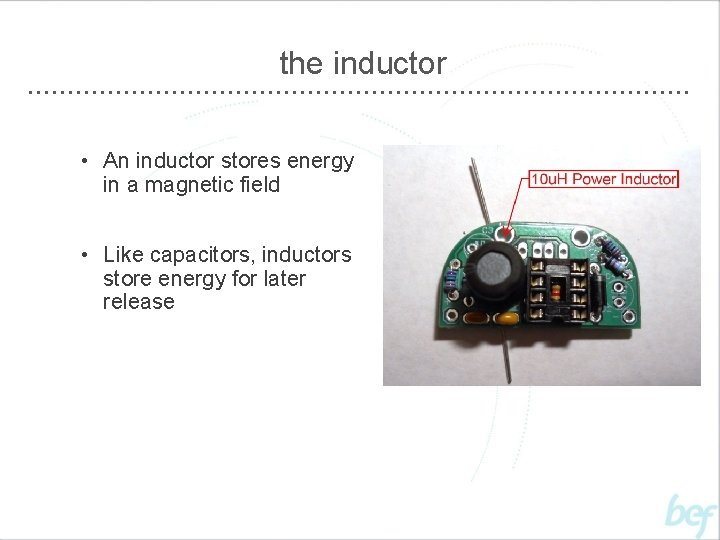the inductor • An inductor stores energy in a magnetic field • Like capacitors, inductors store energy for later release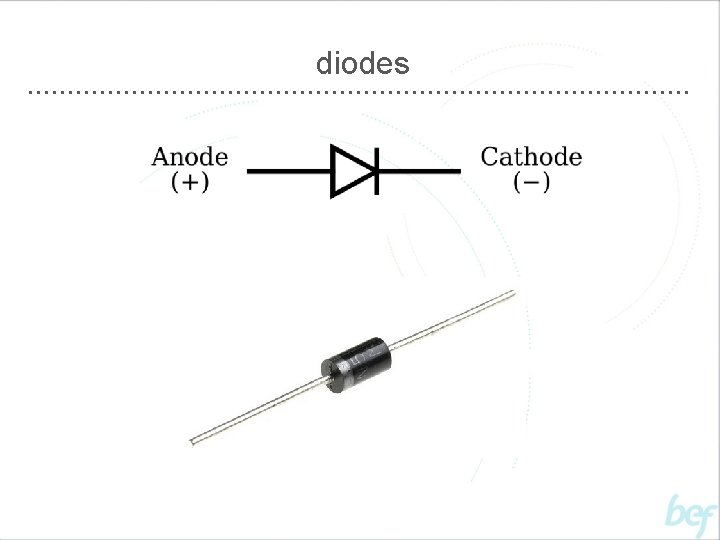diodes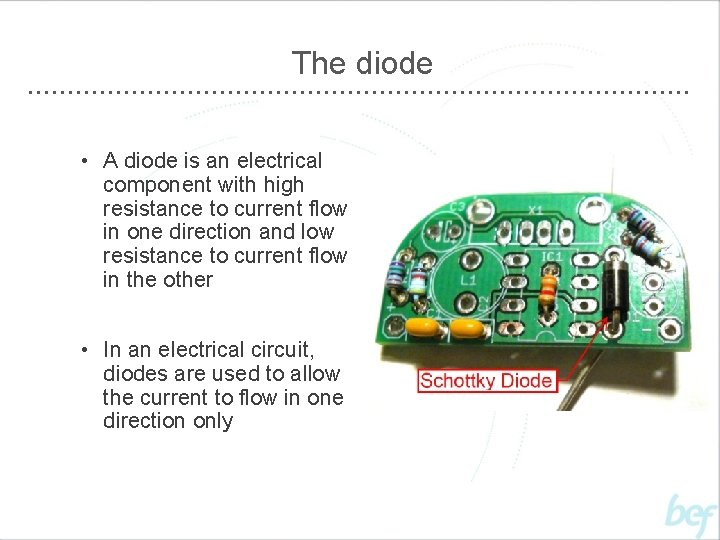The diode • A diode is an electrical component with high resistance to current flow in one direction and low resistance to current flow in the other • In an electrical circuit, diodes are used to allow the current to flow in one direction only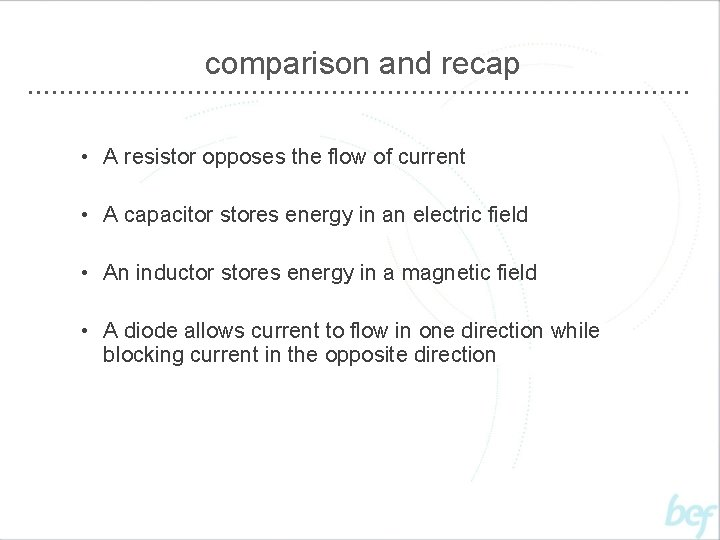comparison and recap • A resistor opposes the flow of current • A capacitor stores energy in an electric field • An inductor stores energy in a magnetic field • A diode allows current to flow in one direction while blocking current in the opposite direction